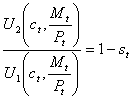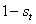# Prof. Bryan Caplan

bcaplan@gmu.edu

http://www.gmu.edu/departments/economics/bcaplan

Econ 816

Spring, 2000

HW#1

Part 1: Mathematical Problems

1.  Romer, problem 9.2.

2.  Suppose that.

a.  Apply the formulato this particular utility function to get an equation for money demand as a function of.

b.  Take the log of both sides of the resulting equation.  Derive an equation for (log) nominal money holdings as a function of everything else.

c.  Does your final equation in (b) make sense?

Part 2: Analytical Problems

TYPE succinct (1 page or less!) answers to each of the following:

1.  White: chapter 1, #7

2.  Is involuntary unemployment a coherent concept?  Critique the arguments of one of the following: Lucas, Carmichael, Shapiro and Stiglitz, or Summers (from the week 1 readings).